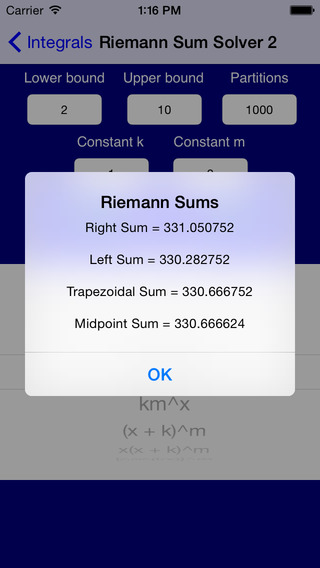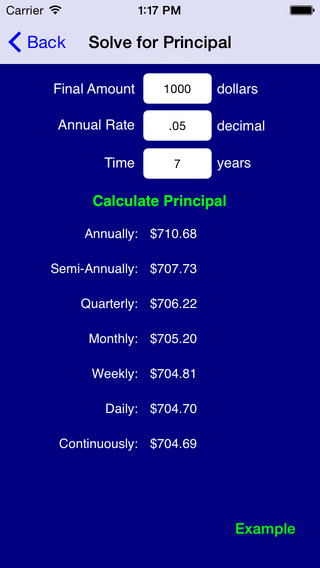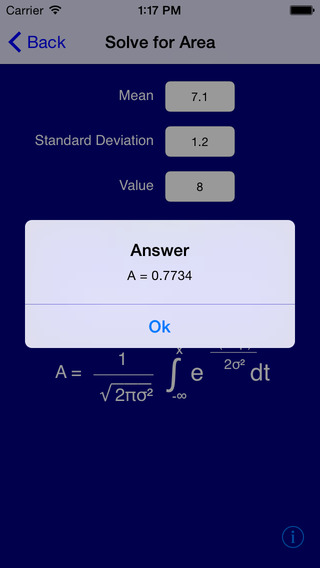Get six applications for the price of two and ace your math classes.

Rated 5/5 by AppleBitesize: www.applebitesize.com/posts/math-pro-review

"Math Pro" will take you through high-school Math and beyond. It is a powerful tool that is overflowing with the tutorials, examples, and solvers from the following applications: Algebra Pro, Geometry Pro, Probability Pro, Statistics Pro, PreCalculus Pro, and Calculus Pro.

"Math Pro" is completely searchable, and it covers an astonishing array of topics:

- Classifying Numbers
- Points and Lines
- Systems of Equations
- Word Problems
- Inequalities
- Linear Programming
- Direct and Inverse Variation
- Polynomials
- Zeros and End Behavior
- Synthetic Division
- Remainder Theorem
- Factoring
- Domains and Ranges
- Compositions of Functions
- Function Transformations
- Inverse Functions
- Piecewise Functions
- Quadratic Functions
- Quadratic Formula
- Perimeter, Area and Volume
- Angles
- Segments
- Midpoints
- Distance Formula
- Distance from Point to Line
- Law of Sines
- Law of Cosines
- Solving Triangles
- Similar Triangles
- Congruent Triangles
- 30-60-90 Triangles
- 45-45-90 Triangles
- Medians
- Altitudes
- Perpendicular Bisectors
- Orthocenters
- Circumcenters
- Centroids
- Circles
- Quadrilaterals
- Regular Polygons
- Logic Statements
- Postulates and Theorems
- Geometric Proofs
- Venn Diagrams
- Bayes' Theorem
- Survey Results
- Odds
- Permutations
- Combinations
- Taking Balls from Urns
- Replacement vs. Non-Replacement
- Independent Events
- Dice
- Roulette
- Lottery
- Poker
- General Card Problems
- Expected Value
- Birthday Problem
- Five Number Summaries
- Outliers
- Linear Regression
- Chebyshev's Theorem
- Normal Distributions
- Binomial Distributions
- Poission Distributions
- Exponential Distributions
- Chi Squared Distributions
- T-Tests
- Confidence intervals
- Uniform Random Number Generator
- Binomial Random Number Generator
- Gaussian Random Number Generator
- Poisson Random Number Generator
- Exponential Random Number Generator
- Logarithms
- Logarithmic Functions
- Exponential Functions
- Rational Functions
- Compound Interest
- Graph Symmetry
- Conic Sections
- Arithmetic Sequences
- Geometric Sequences
- Matrices
- Binomial Expansion
- Complex Numbers
- Rectangular and Polar Notation
- Standard Unit Circle
- Unit Circle Representations of the Six Trig Functions
- Attributes and Graphical Representations of the Six Trig Functions
- Changes in Amplitude, Period, Horizontal Shifts and Vertical Shifts
- Formulas and Identities
- Degree/Radian Conversions
- Inverse Trig Functions
- Reference Angles
- Simplifying Trig Expressions
- Angles of Elevation and Depression
- Polar curves: Limacons, Rose Curves, and Lemniscates
- Limits
- Continuity
- Differentiability
- RDQ, LDQ, SDQ and NDQ
- Equations of Tangent Lines
- Partial Derivatives
- Implicit Differentiation
- Chain Rule
- Product Rule
- Quotient Rule
- Related Rates Problems
- Optimization Examples (Min/Max)
- Derivatives of Inverse Functions
- Taylor and Maclaurin Series
- Derivative Tables
- Derivatives of Integrals
- Arc Length
- Riemann Sums
- Fluid Problems
- Double Integrals
- Integration by Parts
- Definite and Indefinite Integrals
- Volumes of Solids of Revolution
- Cross Sectional Volumes
- Integral tables
- Position, Velocity and Acceleration
- Calculus Theorems

I welcome all comments, questions, and suggestions: lfeldman65@gmail.com

I will answer your math questions for free on Facebook:

www.facebook.com/lfeldmantutoring